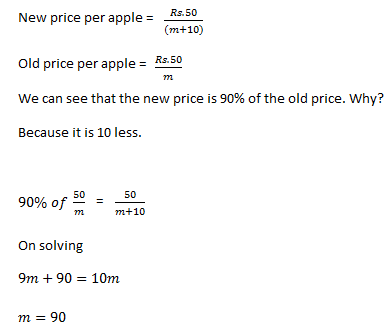New Students Offer - Use Code HELLO

# Percentages Tricks - Application with Examples

Few weeks back I shared how to make application of Percentages concept. Today I am sharing examples to illustrate the usage of this concept.#### Question 1.

Salary of A is 75% of B’s salary. Salary of A is increased by 40% but salary of B is increased by only 10%. Whose salary is more and by what percent?
Solution:

Salary of A = 75% of Salary of B#### Question 2.

The price of a commodity increases by 25% and therefore a house wife reduces the quantity purchased by certain percentage in order to maintain expenditure. By what percentage should she reduce the quantity purchased ?The short cut could be made on the basis that

Price ∝  1/Quantity

Which means if you reduce the price , the quantity will increase and vice-versa, so our formula would be :#### Question 3.

The price of a commodity increases by 25%. Find out the reduction in quantity such that the expenditure is allowed to increase by 20%?

Now again let the price = 100, Quantity =100

Expenditure =100 X 100= 10000

New Price =125, New Quantity =?

New Expenditure =10000+20%of 10000=12000

New Expenditure= New Price X New Quantity

12000 =   125 X New Quantity

New Quantity = 12000/125= 96
Required reduction% in the quantity = (100-96)/100 X 100 =4%

#### Question 4.

The price of a commodity increases by 20% and expenditure is allowed to increase by 15%. What changes would occur in the quantity ?#### Question 5.

The price of an apple reduce by 10% and thus enables a person to buy 10 more apples for Rs. 50. Find out :
1. New price
2. Old Price
3. New Quantity
4. Old Quantity
Solution:

Let us say, initially he is able to buy m apples for Rs.50.
Initially

Rs.50    = m apples

Now

Rs. 50 = (m+10) applesNow we can see that

⇒ Earlier in Rs. 50 , 90 apples could be bought

Now in Rs. 50, 90+10=100 apples could be bought. which clearly shows that the price has dropped

New price = Rs. 50/100

Old price = Rs. 50/90

Now let us learn a quick way

The person has got = Rs 50

The price reduces by 10 % that means he could save 10% of what he has in his pocket.

He could buy the apples in Rs 45 and can save Rs 5,  but instead of saving, he is buying 10 apples for Rs 5

⇒ Rs. 5  = 10 apples

Rs. 1  = 2 apples which means Rs 50 = 100 apples

The new price of one apple = Rs.0.5

Earlier Rs 50 = 100-10 = 90 apples

The old price per apple = 50/90= Rs. 0.55

So this was another method to deal with such questions.

#### Question 6.

A container has got 130 liters of water which has 15% salt. From this container some of the water has got evaporated and after evaporation, the salt strength in the container is now 20%. Find out how much water evaporated from the container.
Solution - Let the water evaporated= m liter